# How to solve (solution) Google's Blockly Future Programmers Game: Turtle Level

Google's Blockly Games is a series of educational games that teach programming. It is based on the Blockly library. All code is free and open source. The Turtle Level aims to dive you deep into loops. Use nested loops to paint multiple shapes and pictures. The game engine and source is available as an open source project at Github here.

In this article we'll share with you the solution to all 9 levels available in the Turtle Game of Blockly.

## Level #1

Create a program that draws a square.The respective JavaScript interpretation of the first level is:

``````for (var count = 0; count < 4; count++) {
moveForward(100);
turnRight(90);
}``````

## Level #2

Change your program to draw a pentagon instead of a square.The respective JavaScript interpretation of the second level is:

``````for (var count = 0; count < 5; count++) {
moveForward(100);
turnRight(72);
}``````

## Level #3

There's a new block that allows you to change the colour, draw a yellow star.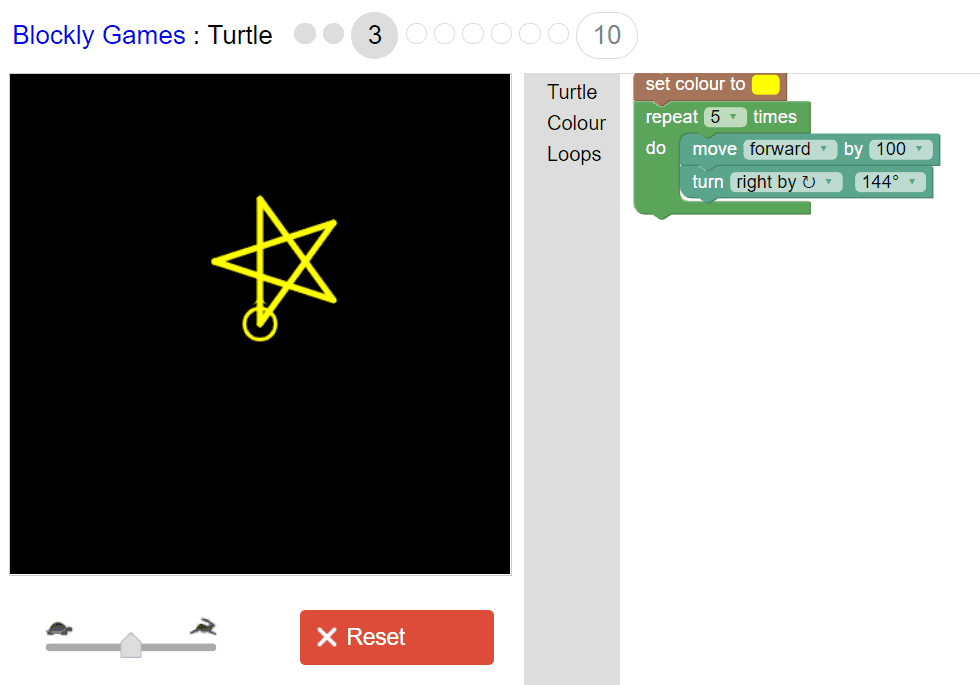The respective JavaScript interpretation of the third level is:

``````penColour('#ffff00');

for (var count = 0; count < 5; count++) {
moveForward(100);
turnRight(144);
}``````

## Level #4

There's a new block that allows you to lift your pen off the paper when you move. Draw a small yellow star, then draw a line above it.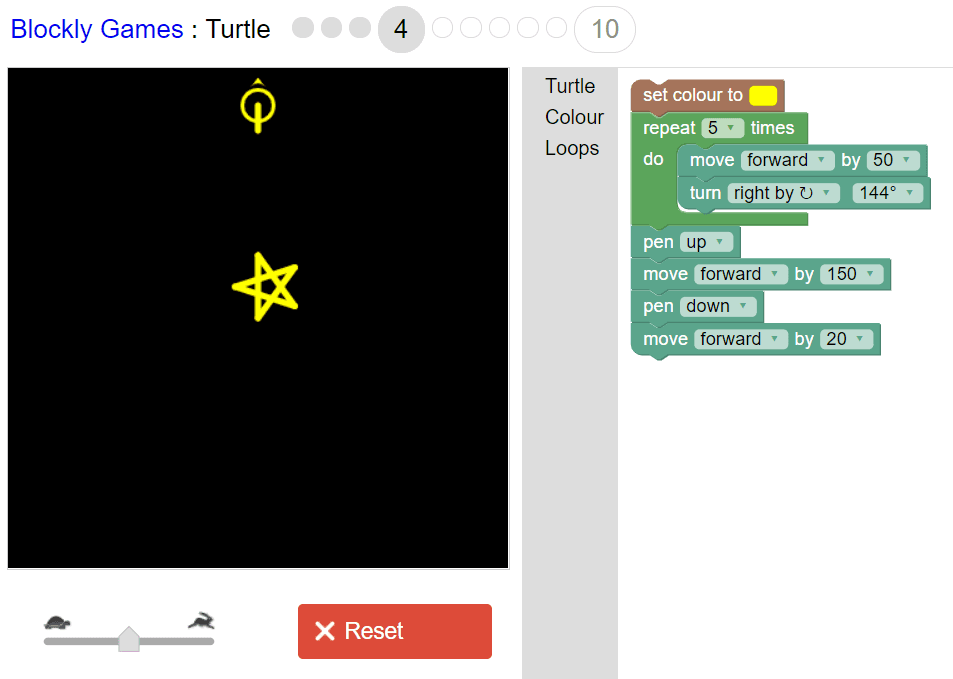The respective JavaScript code for the fourth block is:

``````penColour('#ffff00');

for (var count = 0; count < 5; count++) {
moveForward(50);
turnRight(144);
}

penUp();
moveForward(150);
penDown();
moveForward(20);``````

## Level #5

Instead of one star, can you draw four stars arranged in a square?The respective JavaScript code for the fifth block is:

``````penColour('#ffff00');
for (var count = 0; count < 5; count++) {
moveForward(50);
turnRight(144);
}
penUp();
moveForward(150);
penDown();
turnRight(90);
for (var count2 = 0; count2 < 5; count2++) {
moveForward(50);
turnRight(144);
}
penUp();
moveForward(150);
penDown();
turnRight(90);
for (var count3 = 0; count3 < 5; count3++) {
moveForward(50);
turnRight(144);
}
penUp();
moveForward(150);
penDown();
turnRight(90);
for (var count4 = 0; count4 < 5; count4++) {
moveForward(50);
turnRight(144);
}``````

## Level #6

Draw the stars, then draw the line.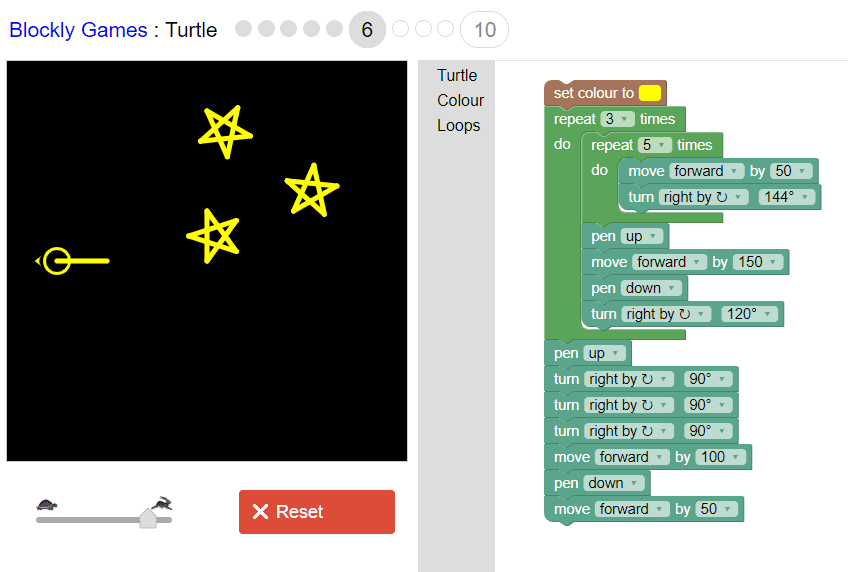The respective JavaScript code for the sixth block is:

``````penColour('#ffff00');
for (var count2 = 0; count2 < 3; count2++) {
for (var count = 0; count < 5; count++) {
moveForward(50);
turnRight(144);
}
penUp();
moveForward(150);
penDown();
turnRight(120);
}
penUp();
turnRight(90);
turnRight(90);
turnRight(90);
moveForward(100);
penDown();
moveForward(50);``````

## Level #7

Draw the stars, then draw four white lines.The respective JavaScript code for the seventh block is:

``````penColour('#ffff00');
for (var count2 = 0; count2 < 3; count2++) {
for (var count = 0; count < 5; count++) {
moveForward(50);
turnRight(144);
}
penUp();
moveForward(150);
penDown();
turnRight(120);
}
penUp();
for (var count3 = 0; count3 < 3; count3++) {
turnRight(90);
}
moveForward(100);
penDown();
for (var count4 = 0; count4 < 3; count4++) {
turnRight(45);
}
moveForward(50);
for (var count6 = 0; count6 < 3; count6++) {
penUp();
turnRight(90);
turnRight(90);
moveForward(50);
penDown();
for (var count5 = 0; count5 < 3; count5++) {
turnRight(45);
}
moveForward(50);
}``````

## Level #8

Drawing 360 white lines will look like the full moon.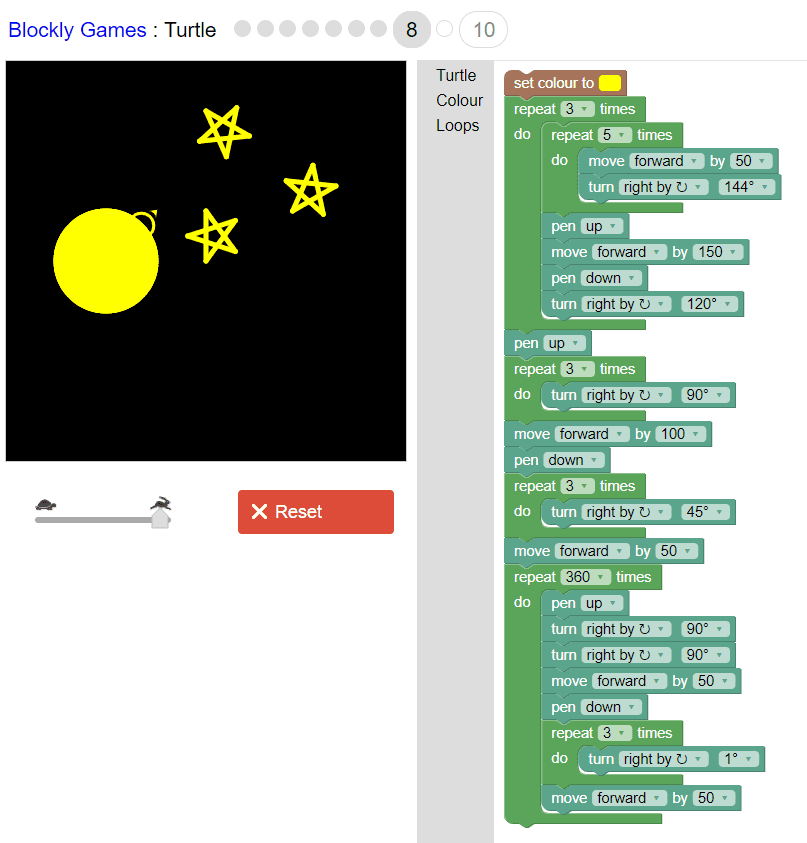The respective JavaScript code for the eighth block is:

``````penColour('#ffff00');
for (var count2 = 0; count2 < 3; count2++) {
for (var count = 0; count < 5; count++) {
moveForward(50);
turnRight(144);
}
penUp();
moveForward(150);
penDown();
turnRight(120);
}
penUp();
for (var count3 = 0; count3 < 3; count3++) {
turnRight(90);
}
moveForward(100);
penDown();
for (var count4 = 0; count4 < 3; count4++) {
turnRight(45);
}
moveForward(50);
for (var count6 = 0; count6 < 360; count6++) {
penUp();
turnRight(90);
turnRight(90);
moveForward(50);
penDown();
for (var count5 = 0; count5 < 3; count5++) {
turnRight(1);
}
moveForward(50);
}``````

## Level #9

Can you add a black circle so that the moon becomes a crescent?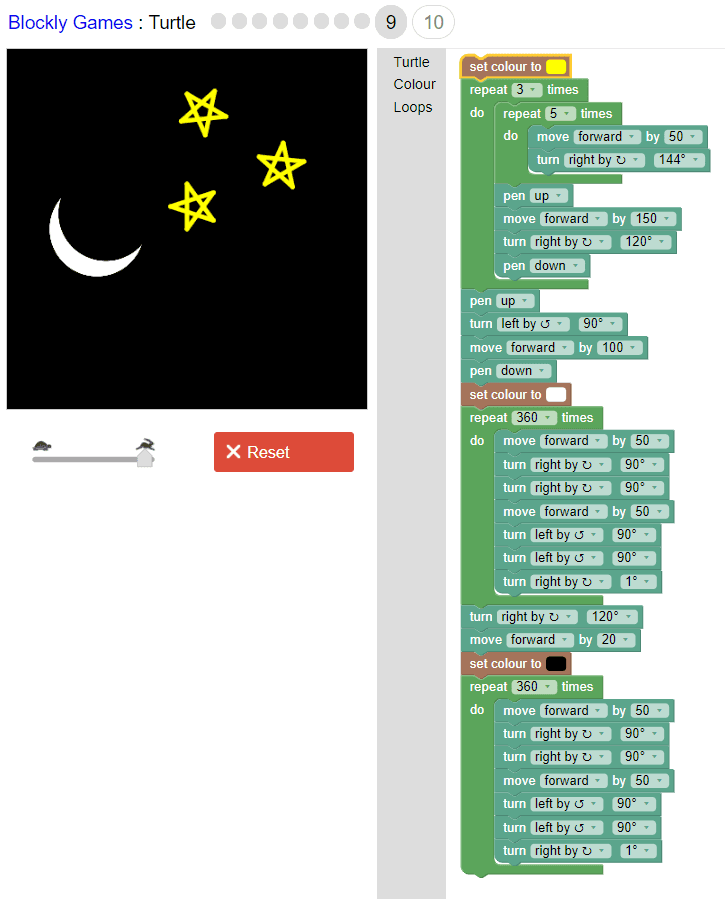The respective JavaScript code for the ninth block is:

``````penColour('#ffff00');
for (var count2 = 0; count2 < 3; count2++) {
for (var count = 0; count < 5; count++) {
moveForward(50);
turnRight(144);
}
penUp();
moveForward(150);
turnRight(120);
penDown();
}
penUp();
turnLeft(90);
moveForward(100);
penDown();
penColour('#ffffff');
for (var count3 = 0; count3 < 360; count3++) {
moveForward(50);
turnRight(90);
turnRight(90);
moveForward(50);
turnLeft(90);
turnLeft(90);
turnRight(1);
}
turnRight(120);
moveForward(20);
penColour('#000000');
for (var count4 = 0; count4 < 360; count4++) {
moveForward(50);
turnRight(90);
turnRight(90);
moveForward(50);
turnLeft(90);
turnLeft(90);
turnRight(1);
}``````

Happy coding !